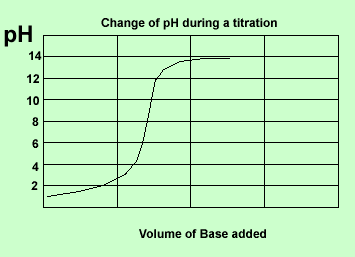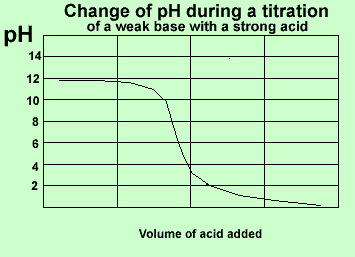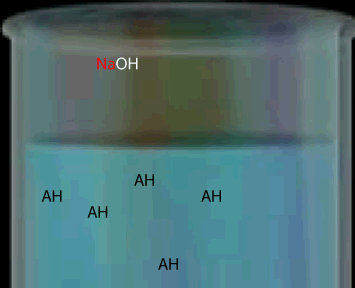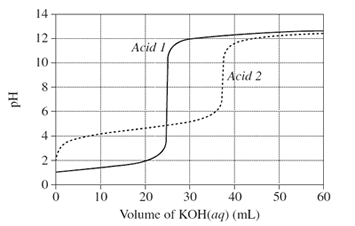Volumetric analysis There are many instances where we need to find the amount of acid or base present in a given volume of liquid. Volumetric analysis is a technique of finding unknown concentrations. This involves mixing two solutions, one with a known concentration and the other with the unknown concentration. The solutions are mixed until they have just reacted completely. This procedure is known as a titration. The point at which the solutions are mixed in just the right stoichiometric ratio to achieve complete reaction is called the equivalence point. Since the solutions used are often clear, indicators are used to identify the time during the reaction when neutralisation is achieved(end point). Indicators change colour at different pH levels and this alerts us to the fact that we are at the end point. In order to determine the unknown concentration we need two things: - a balanced chemical equation of the reaction between the reactants in the two solutions. - the accurate value of the average titre. A titre is the volume of solution, delivered by a burette, that is needed to reach the end point.The pH profile on the left is that of a neutralisation reaction. An acid is slowly titrated with a strong base. The pH slowly rises as base is added. The profiles change as different strength acids and bases are used. The pH profile on the left is that of a weak base titrated against a strong acid.Here is a summary of the curves. Strong acid vs weak base Strong base vs weak acid Weak acid vs strong base Weak base vs weak acid Strong acid vs strong baseNote that the equivalence point is not always at a pH of 7. The equivalence point depends on the strength of the acid or base being titrated. Consider a weak monoprotic acid titrated against a strong base such as NaOH. At the equivalence point all the acid has reacted leaving only the conjugate base. A weak acid has a strong conjugate base so the solution remaining is some what basic. Hence the equivalence point will be above pH 7.The graph, on the right, shows changes in pH for the titrations of equal volumes of solutions of two monoprotic acids, Acid 1 and Acid 2.Explain the differences between Acid 1 and Acid 2 in terms of their relative strengths and concentrations. SolutionContinue

Home